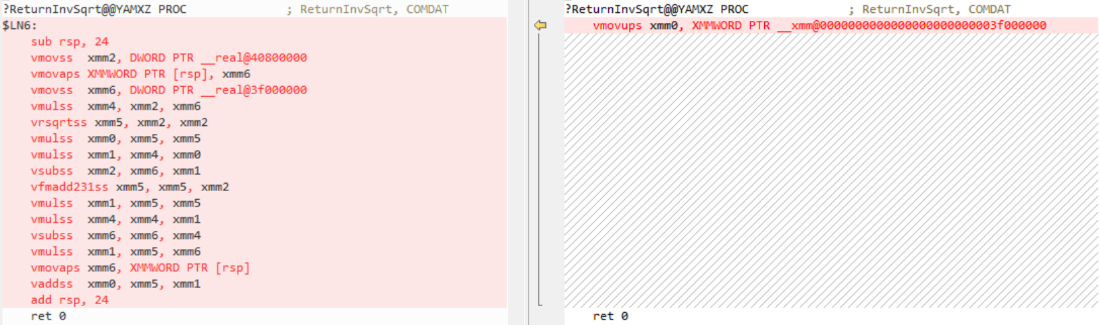# AVX2 floating point improvements in Visual Studio 2019 version 16.5Bran

In Visual Studio 2019 We’ve been working hard on optimizing floating point operations with AVX2 instructions. This post will outline work done so far and recent improvements made in version 16.5.

The speed of floating point operations directly impacts the frame rate of video games. Newer x86 and x64 chips have added special vector Fused Multiply Add instructions to improve and parallelize the performance of floating point operations. Starting with Visual Studio 2019, the compiler will aggressively identify opportunities to use the new floating point instructions and perform constant propagation for such instructions when the /fp:fast flag is passed.

With Visual Studio 2019 version 16.2, the heuristics for vectorizing floating point operations improved and some floating point operations could be reduced down to a constant. Natalia Glagoleva described these and a number of game performance improvements last summer.

With Visual Studio 2019 version 16.5, we improved the SSA optimizer to recognize more opportunities to use AVX2 instructions and improved constant propagation for vector operations involving shuffle.

All of the following samples are compiled for x64 with these switches: /arch:AVX2 /O2 /fp:fast /c /Fa

### Constant Propagation for Multiply

Starting with Visual Studio 2019 version 16.2, some floating point vector operations could be reduced to a constant if the initial vectors were known at compile time. A good example is the inverse square root function.

```#include
#include
float InvSqrt(float F)
{
const __m128 fOneHalf = _mm_set_ss(0.5f);
__m128 Y0, X0, X1, X2, FOver2;
float temp;
Y0 = _mm_set_ss(F);
X0 = _mm_rsqrt_ss(Y0);
FOver2 = _mm_mul_ss(Y0, fOneHalf);
X1 = _mm_mul_ss(X0, X0);
X1 = _mm_sub_ss(fOneHalf, _mm_mul_ss(FOver2, X1));
X2 = _mm_mul_ss(X1, X1);
X2 = _mm_sub_ss(fOneHalf, _mm_mul_ss(FOver2, X2));
_mm_store_ss(&temp, X2);
return temp;
}

float ReturnInvSqrt()
{
return InvSqrt(4.0);
}```

Starting with Visual Studio 16.2, ReturnInvSqrt could be reduced to a single constant:### Constant Propagation for Shuffle

Another common vector operation is to create a normalized form of the vector, so that it has a length of one. The length of a vector is the square root of its dot product. The easiest way to calculate the dot product involves a shuffle operation.

```__m128  VectorDot4(const __m128 Vec1, const __m128 Vec2)
{
__m128 Temp1, Temp2;
Temp1 = _mm_mul_ps(Vec1, Vec2);
Temp2 = _mm_shuffle_ps(Temp1, Temp1, 0x4E);
Temp2 = _mm_shuffle_ps(Temp1, Temp1, 0x39);
}

__m128  VectorNormalize_InvSqrt(const __m128 V)
{
const __m128 Len = VectorDot4(V, V);
const float LenComponent = ((float*) &Len);
const float rlen = InvSqrt(LenComponent);
}```

Even in Visual Studio version 16.0 the optimizer could propagate constants through shuffle operations. However, due to some ordering issues with the original implementation of fused multiply add constant propagation, constant propagation for shuffle prevented constant propagation for fused multiply add.

Starting with Visual Studio 16.5, constant propagation can handle cases that involve both shuffle and fused multiply add. This means normalizing the inverse square root of a vector known at compile time can be completely reduced down to a constant if the input is known at compile time.

```__m128 ReturnVectorNormalize_InvSqrt() {
__m128 V0 = _mm_setr_ps(2.0f, -2.0f, 2.0f, -2.0f);
return VectorNormalize_InvSqrt(V0);
}```We’d love for you to download the latest version of Visual Studio 2019 and give these new improvements a try. As always, we welcome your feedback. We can be reached via the comments below or via email (visualcpp@microsoft.com). If you encounter problems with Visual Studio or MSVC, or have a suggestion for us, please let us know through Help > Send Feedback > Report A Problem / Provide a Suggestion in the product, or via Developer Community. You can also find us on Twitter (@VisualC).